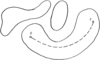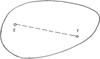International
Tables for
Crystallography
Volume A
Space-group symmetry
Edited by Th. Hahn

International Tables for Crystallography (2006). Vol. A. ch. 9.3, pp. 756-757

## Section 9.3.2. Topological characteristic of lattice characters

B. Grubera

aDepartment of Applied Mathematics, Faculty of Mathematics and Physics, Charles University, Malostranské nám. 25, CZ-11800 Prague 1, Czech Republic

### 9.3.2. Topological characteristic of lattice characters

| top | pdf |

In his thorough analysis of lattice characters, de Wolff (1988)remarks that so far they have not been defined as clearly as the Bravais types and that an exact general definition does not exist. Gruber (1992)tried to base such a definition on topological concepts.

The crucial notion is the decomposition of a set M of points of the n-dimensional Euclidean spaceinto equivalence classes called components of the set M. They can be defined as follows: Two points X,Y of the set M belong to the same component if they can be connected by a continuous path which lies entirely in the set M (Fig. 9.3.2.1). This partition of the set M into components is unique and is determined solely by the set M.Figure 9.3.2.1 | top | pdf |A setconsisting of three components.

Now let us return to lattices. To any lattice L there is attached a point incalled the Niggli point of L. It is the pointprovided that the vectors a, b, c describe the Niggli cell of L and fulfil the conditions (9.2.2.2a) to (9.2.2.5f) of Section 9.2.2. Ifis a set of lattices then the set of Niggli points of all lattices ofis called the Niggli image of.

Thus we can speak about the Niggli image of a Bravais type. This Niggli image is a part ofand so can be partitioned into components. This division of Niggli points induces back a division of lattices of the Bravais type. It turns out that this division is identical with the division ofinto lattice characters as introduced in Section 9.2.5. This fact, used conversely, can be considered an exact definition of the lattice characters: Two lattices of Bravais typeare said to be of the same lattice character if their Niggli points lie in the same component of the Niggli image of.

We can, of course, also speak about Niggli images of particular lattice characters. According to their definition, these images are connected sets. However, much more can be stated about them: these sets are even convex (Fig. 9.3.2.2). This means that any two points of the Niggli image of a lattice character can be connected by a straight segment lying totally in this Niggli image. From this property, it follows that the lattice characters may be defined also in the following equivalent way:Figure 9.3.2.2 | top | pdf |A convex set in.

We say that two lattices of the same Bravais type belong to the same lattice character if one of them can be deformed into the other in such a way that the Niggli point of the deformed lattice moves linearly from the initial to the final position while the Bravais type of the lattice remains unchanged.

Unlike convexity, nothing can be said whether the Niggli images of lattice characters are open sets (with regard to their dimension) or not. Both cases occur.

The lattice character of a lattice L can also be recognized [instead of by means of Table 9.2.5.1or by Tables 1 and 3 in Gruber (1992)] by perpendicular projection of the c vector onto the ab plane provided the vectors a, b, c describe the Niggli cell of L and fulfil the conditions (9.2.2.2a) to (9.2.2.5f) in Section 9.2.2(de Wolff & Gruber, 1991). See also Figs. 9.2.4.1to 9.2.4.5.

### ReferencesGruber, B. (1992). Topological approach to the Niggli lattice characters. Acta Cryst. A48, 461–470.Google ScholarWolff, P. M. de (1988). Definition of Niggli's lattice characters. Comput. Math. Appl. 16, 487–492.Google ScholarWolff, P. M. de & Gruber, B. (1991). Niggli lattice characters: definition and graphical representation. Acta Cryst. A47, 29–36.Google Scholar